GCSE Maths Probability

# Probability

Here you will find 35 GCSE probability revision guides. Like all our GCSE maths revision topics, we've included step by step resources, detailed examples, practice questions, exam questions and probability worksheets! All GCSE questions are based on actual Edexcel, AQA and OCR exam questions with mark scheme and model answers provided.

Filter by:

How to Calculate Probability

Venn Diagram

Describing Probability

Probability Distribution

Probability

Combined Events

Probability Tree

Frequency Trees

Show all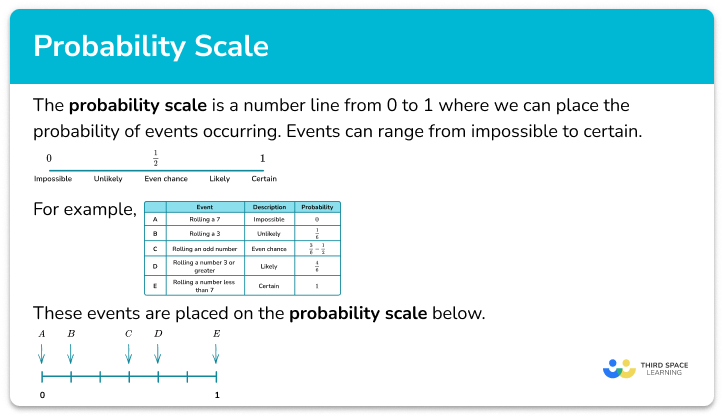Probability scale

Step by step guide, detailed examples, practice questions, exam questions and probability scale worksheets.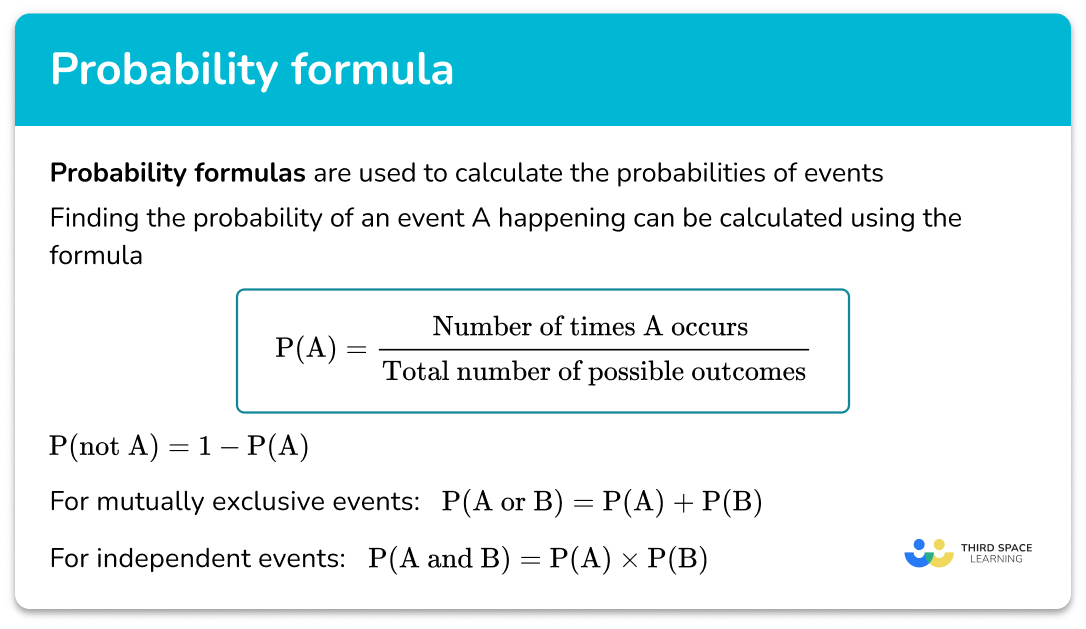Probability formula

Step by step guide, detailed examples, practice questions, exam questions and probability formula worksheets.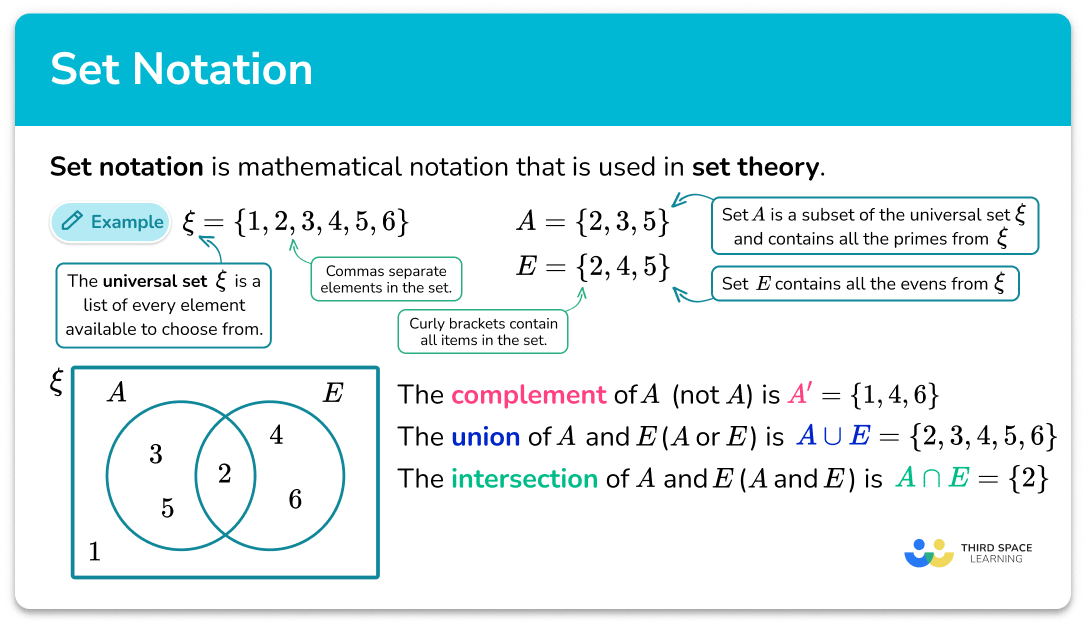Set notation

Step by step guide, detailed examples, practice questions, exam questions and set notation worksheets.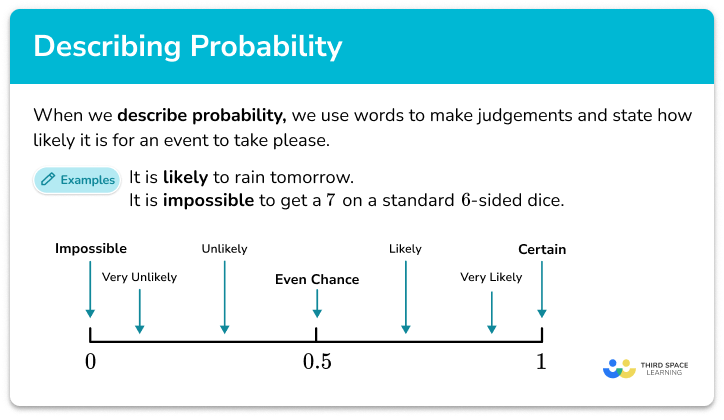Describing probability

Step by step guide, detailed examples, practice questions, exam questions and describing probability worksheets.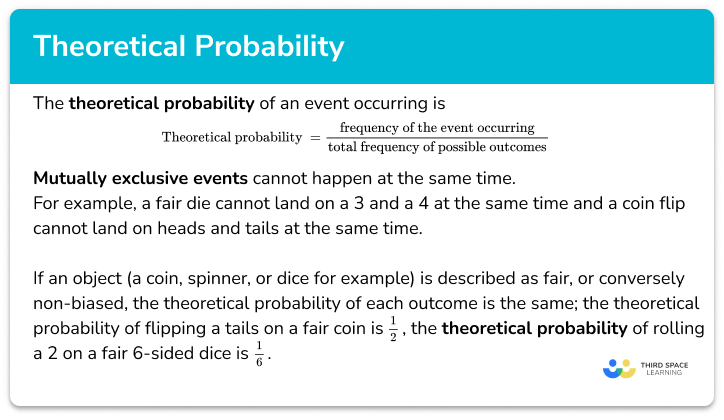Theoretical probability

Step by step guide, detailed examples, practice questions, exam questions and theoretical probability worksheets.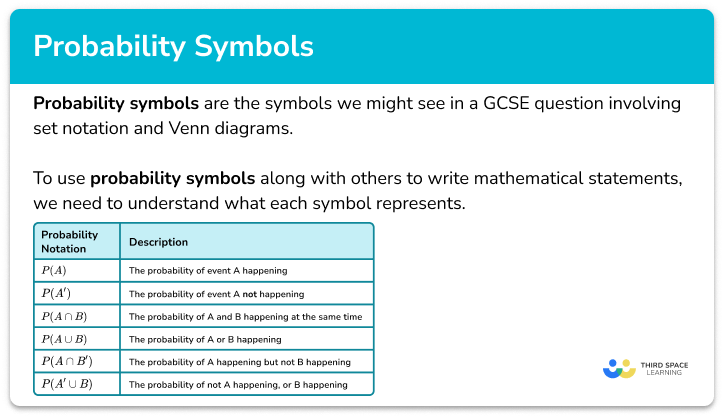Probability symbol

Step by step guide, detailed examples, practice questions, exam questions and probability symbol worksheets.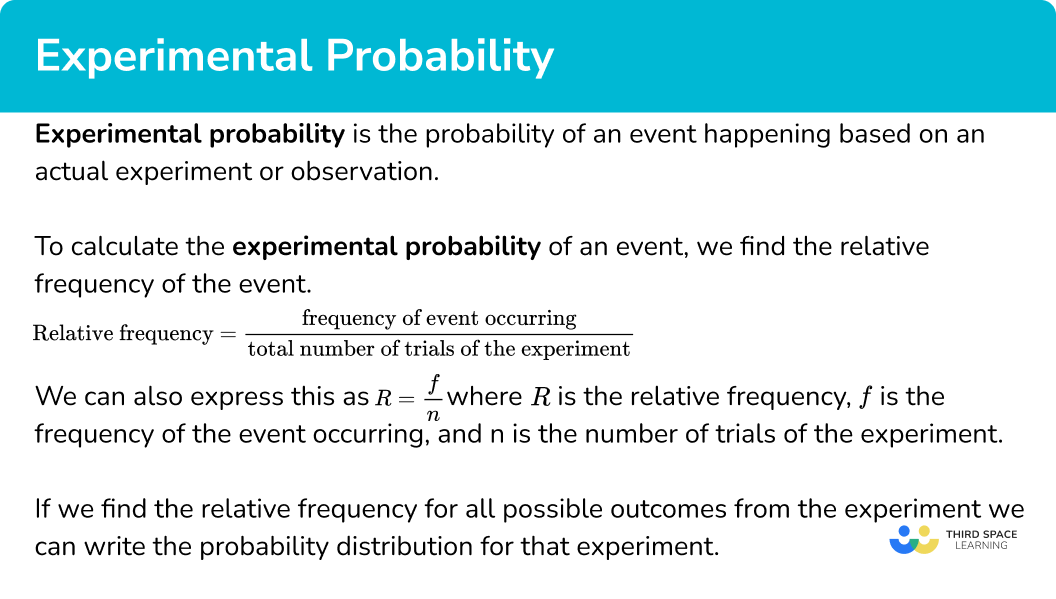Experimental probability

Step by step guide, detailed examples, practice questions, exam questions and experimental probability worksheets.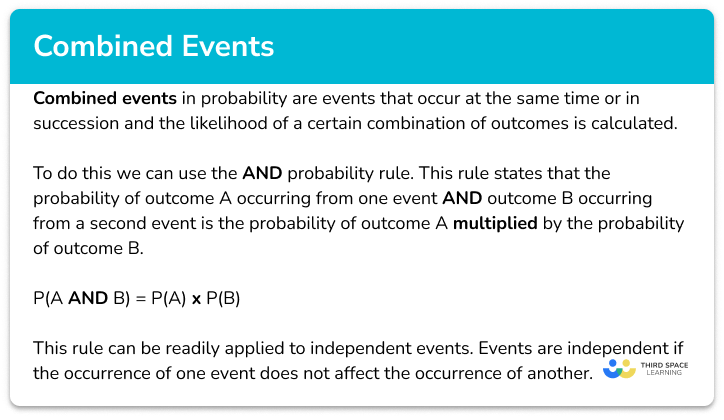Combined events probability

Step by step guide, detailed examples, practice questions, exam questions and combined events probability worksheets.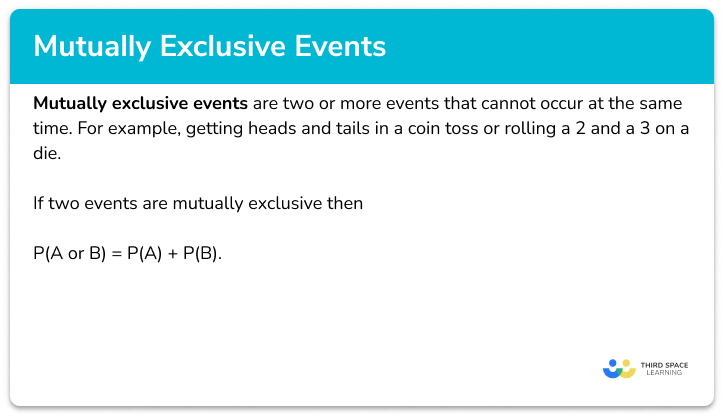Mutually exclusive events

Step by step guide, detailed examples, practice questions, exam questions and mutually exclusive events worksheets.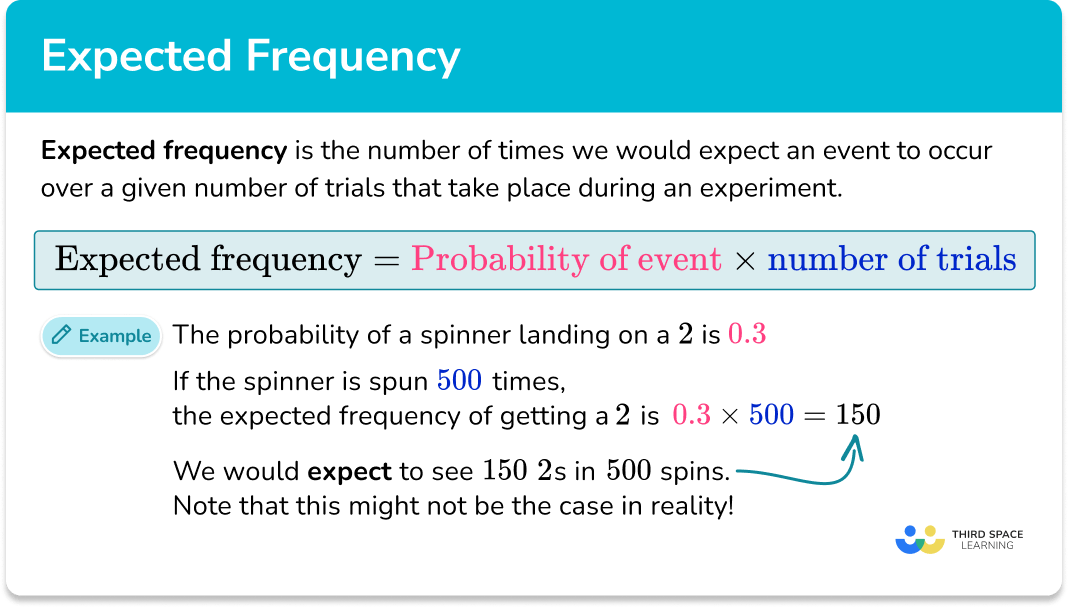Expected frequency

Step by step guide, detailed examples, practice questions, exam questions and expected frequency worksheets.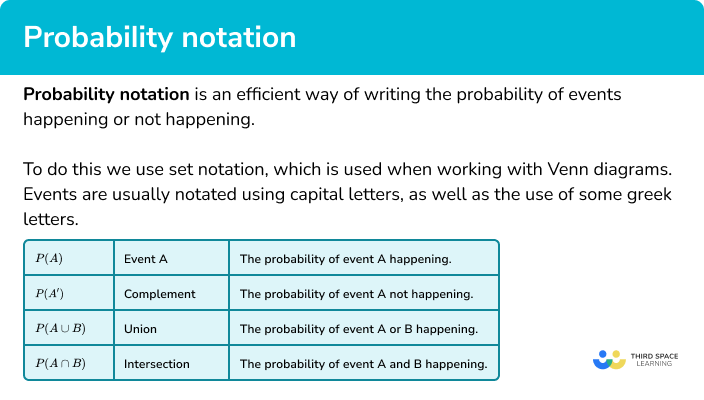Probability notation

Step by step guide, detailed examples, practice questions, exam questions and probability notation worksheets.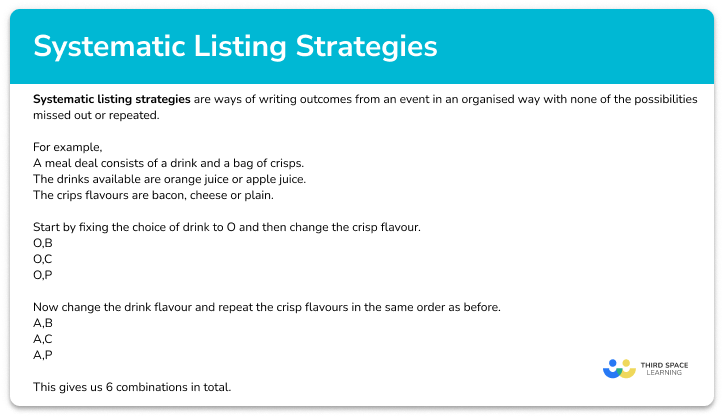Systematic listing strategies

Step by step guide, detailed examples, practice questions, exam questions and systematic listing strategies worksheets.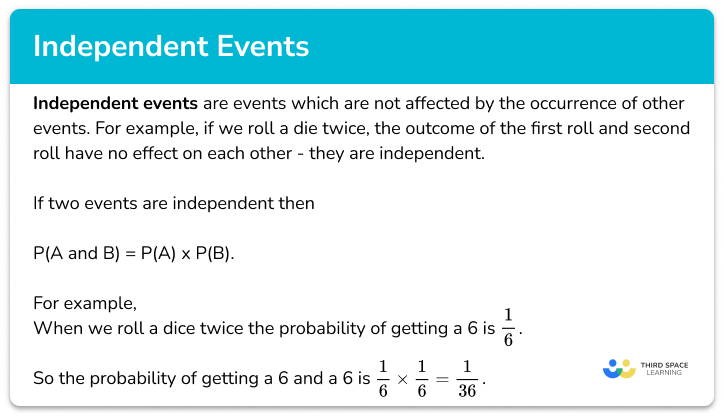Independent events

Step by step guide, detailed examples, practice questions, exam questions and independent events worksheets.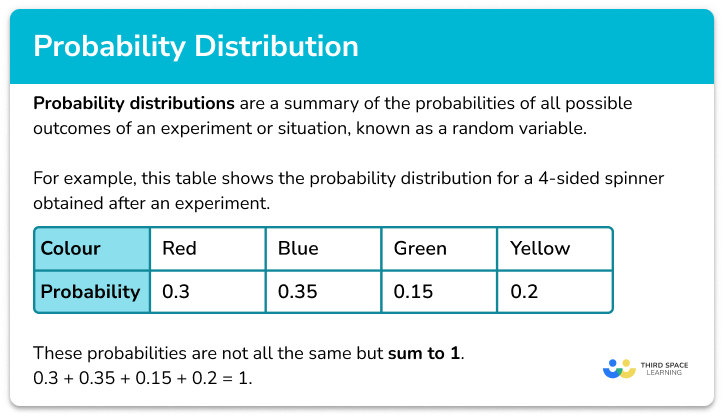Probability distribution

Step by step guide, detailed examples, practice questions, exam questions and probability distribution worksheets.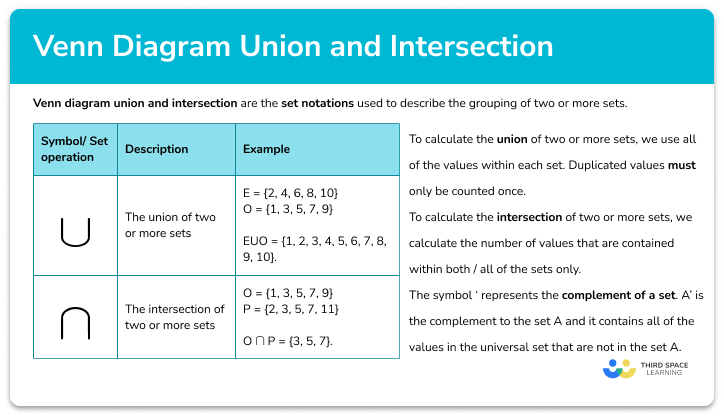Venn diagram union and intersection

Step by step guide, detailed examples, practice questions, exam questions and venn diagram union worksheets.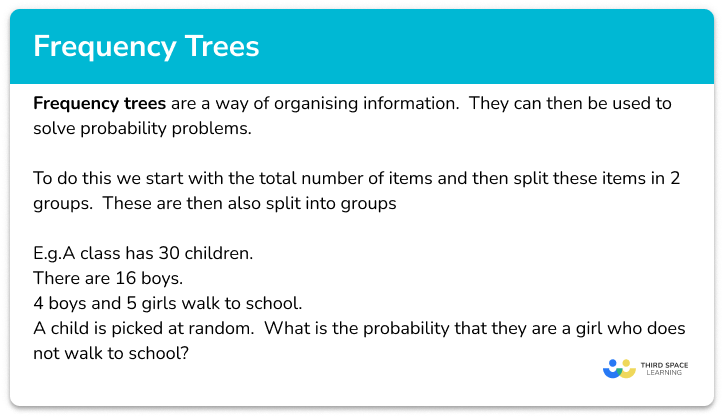Frequency trees

Step by step guide, detailed examples, practice questions, exam questions and frequency trees worksheets.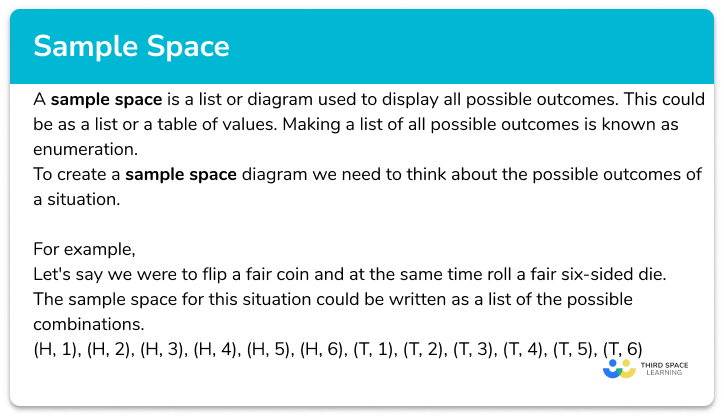Sample space diagram

Step by step guide, detailed examples, practice questions, exam questions and sample space diagram worksheets.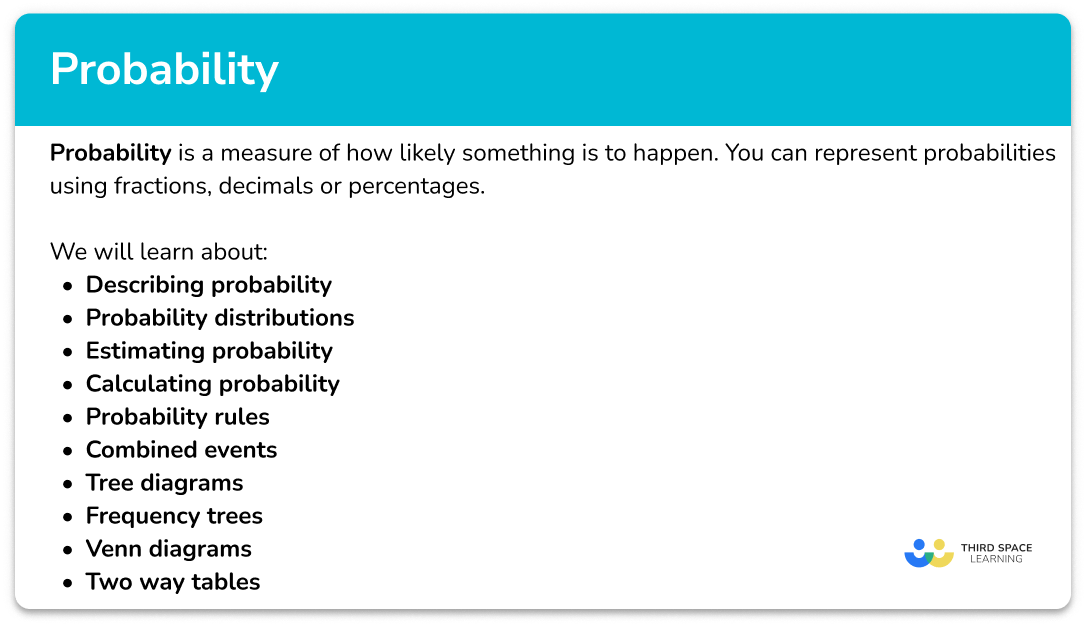Probability – Maths GCSE

Step by step guide, detailed examples, practice questions, exam questions and probability maths worksheets.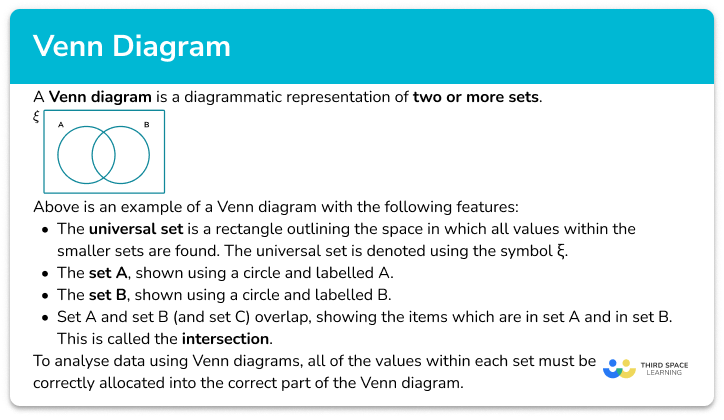Venn diagram

Step by step guide, detailed examples, practice questions, exam questions and Venn diagram worksheets.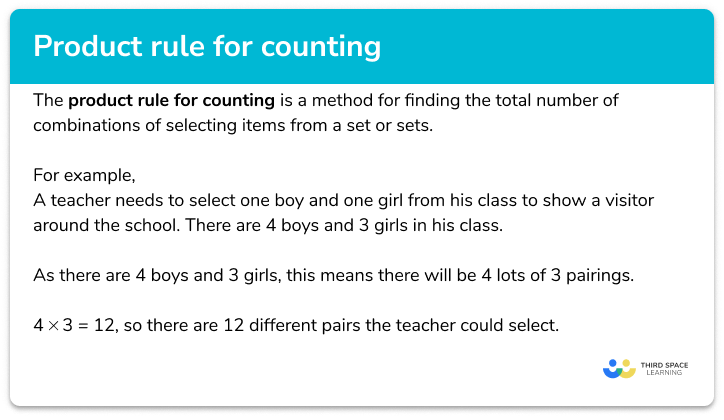Product rule for counting

Step by step guide, detailed examples, practice questions, exam questions and product rule for counting worksheets.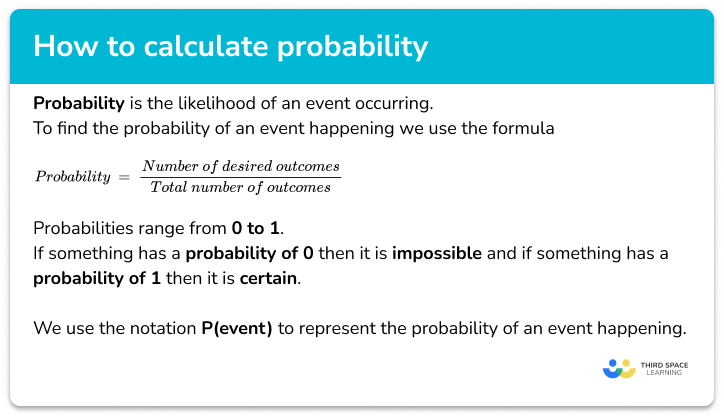How To Calculate Probability

Step by step guide, detailed examples, practice questions, exam questions and how to calculate probability worksheets.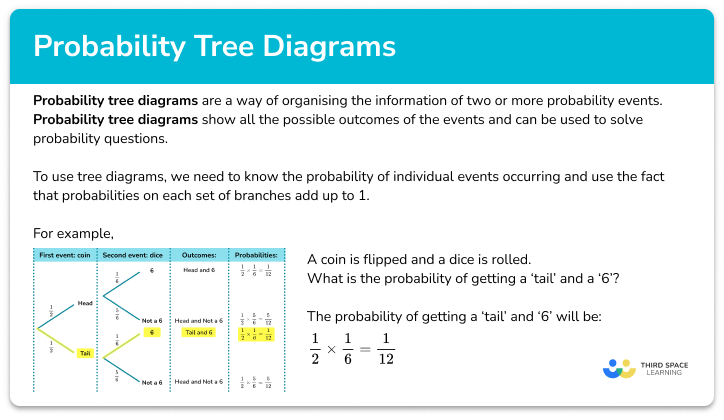Probability tree diagram

Step by step guide, detailed examples, practice questions, exam questions and probability tree worksheets.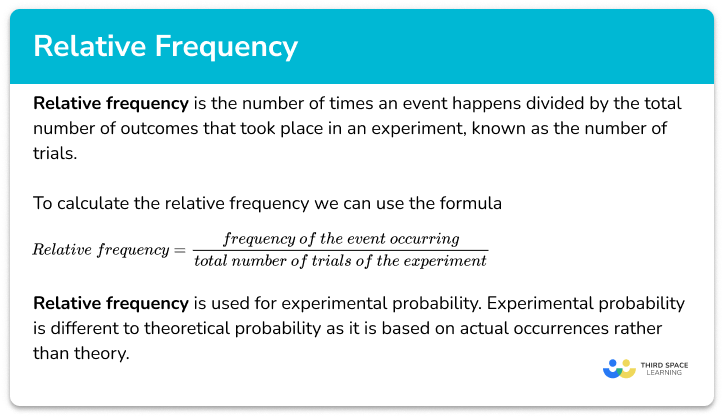Relative frequency

Step by step guide, detailed examples, practice questions, exam questions and relative frequency worksheets.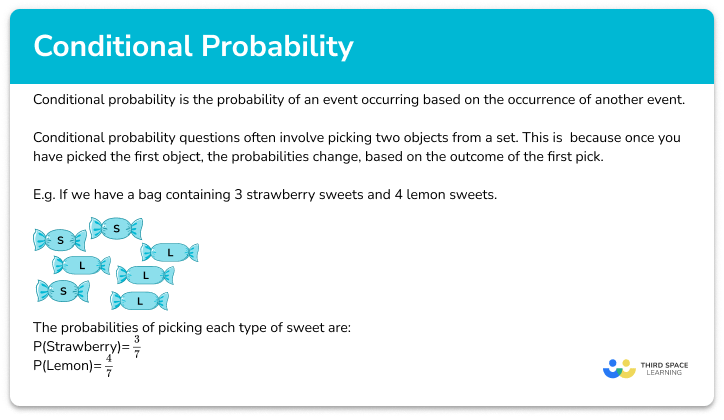Conditional probability

Step by step guide, detailed examples, practice questions, exam questions and conditional probability worksheets.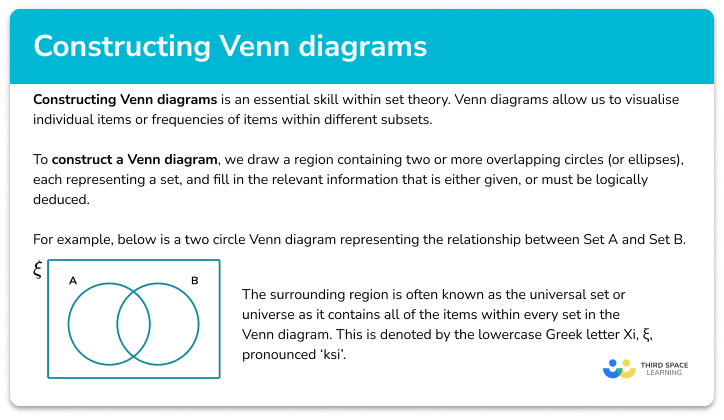Constructing Venn Diagrams

Step by step guide, detailed examples, practice questions, exam questions and constructing Venn diagrams worksheets.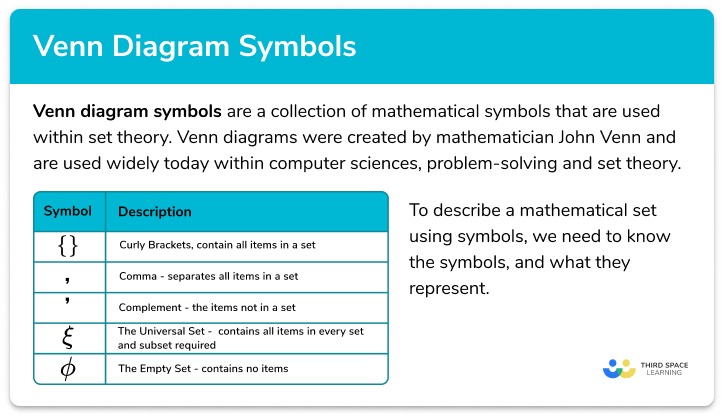Venn diagram symbols

Step by step guide, detailed examples, practice questions, exam questions and Venn diagram symbols worksheets.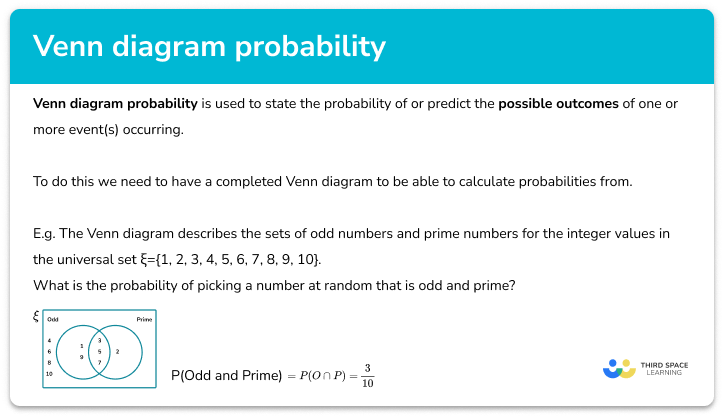Venn Diagram Probability

Step by step guide, detailed examples, practice questions, exam questions and Venn diagram probability worksheets.

One to one maths interventions built for KS4 success

Weekly online one to one GCSE maths revision lessons now available